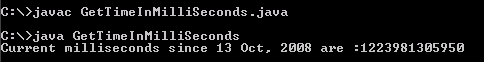Tutorials

# Java Get Time in MilliSeconds

If you wanted to show the time in milliseconds since the particular time, go through the below given example that illustrate how to get the time in milliseconds.

If you wanted to show the time in milliseconds since the particular time, go through the below given example that illustrate how to get the time in milliseconds.

# Java Get Time in MilliSeconds

If you wanted to show the time in milliseconds since the particular time, go through the below given example that illustrate how to get the time in milliseconds. Please do not forget to import the java.util.calender package as it contains all the mechanism to show the time and date etc..

Java Syntax to get time in milliseconds

 ``` import java.util.Calendar; public class GetTimeInMilliSeconds { public static void main(String[] args) { Calendar cal = Calendar.getInstance(); System.out.println("Current milliseconds since 13 Oct, 2008 are :" + cal.getTimeInMillis()); } }```

Output will be displayed as: